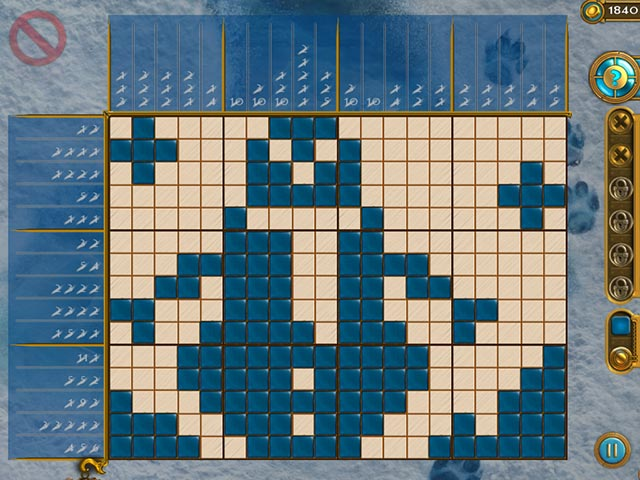Recently I came across a number puzzle called Magic Griddlers from Big Fish. It’s kind of attractive, so I wrote a puzzle solver with several lines of python. It gives the solution in a few seconds.1. Input the patterns of rows and columns. If none of the numbers of a pattern is larger than 9, it can be input as a whole number, e.g. a pattern of ‘1 3 5’ can be input as 135. Otherwise, you should input them as a list. e.g. a pattern of ‘1 3 12’ as [1, 3, 12]
2. Run the python code. Python 3 is required.

Some Notes:
1. intermediate data are saved for calculation performance in savedata func. For the lattices used in the puzzle, only row count or column count is 5, 10, 15, 20 are pre calculated.
2. on the Magic Griddlers page, a reviewer geniegal said: “The puzzles require guesses (or hints). Good puzzles rely on logic to complete without any guess work.”. well, I haven’t played all levels. But I agreed with that “logic only” comment. According to my test, for 2×2 grid dimensions, there exist 2 lattices share a pattern:[‘01’,‘10’] and [‘10’,‘01’].
and for 3×3 grid dimensions, there are 2^(3×3)=512 kinds of lattices, but with only 445 patterns, so it is true some lattices share a pattern. The largest community in 3×3 case is:
[‘001’, ‘010’, ‘100’]
[‘001’, ‘100’, ‘010’]
[‘010’, ‘001’, ‘100’]
[‘010’, ‘100’, ‘001’]
[‘100’, ‘001’, ‘010’]
[‘100’, ‘010’, ‘001’]
these 6 lattices have pattern in common.
So if you want to design a puzzle, you can use my solver to test whether the lattice is a good one. if not, the solver would not find a solution since it is based on logic only.
3. A interesting note of the count of unique patterns of binary sequence, it follows fibonacci numbers as the sequence length increases.